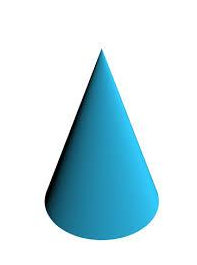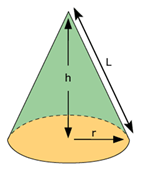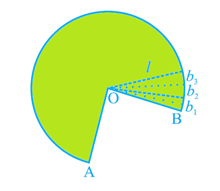# Cone Formula

Cone is a three-dimensional structure having a circular base where a set of line segments, connect all of the points on the base to a common point called apex. A cone can be seen as a set of non-congruent circular discs that are stacked on one another such that ratio of the radius of adjacent discs remains constant. You can think of a cone as a triangle which is being rotated about one of its vertices. There is a predefined set of formulas for the calculation of curved surface area and total surface area of a cone, which is collectively called a cone formula.Curved surface area of a cone =

$$\begin{array}{l}\pi rl\end{array}$$

Total surface area of a cone =

$$\begin{array}{l}\pi r\left ( l+r \right )\end{array}$$

$$\begin{array}{l}l=\sqrt{h^{2}+r^{2}}\end{array}$$

Where, r is the base radius, h is the height and l is the slant height of the cone.

Derivation:

In order to calculate the curved surface area and total surface area of a cone, we divide it into a circular base and the top slanted part. The area of the slanted part gives you the curved surface area. Total surface area is the sum of this circular base and curved surface areas.Area of the circular base:

The base is a simple circle and we know that area of a circle is given as:

Area of a circle=πr²

Where r is the base radius of the cone

Area of the curved surface:

Now if open the curved top and cut into small pieces, so that each cut portion is a small triangle, whose height is the slant height l of the cone.Now the area of each triangle =1/2× base of each triangle × l.

∴Area of the curved surface = sum of the areas of all the triangles

$$\begin{array}{l}=\frac{1}{2}\times b_{1}\times l+ \frac{1}{2}\times b_{2}\times l+\frac{1}{2}\times b_{3}\times l+……… +\frac{1}{2}\times b_{n}\times 1\end{array}$$

$$\begin{array}{l}=\frac{1}{2}l\left ( b_{1}+b_{2}+b_{3}+……+b_{n} \right )\end{array}$$

$$\begin{array}{l}=\frac{1}{2}l\left ( curved surface \right )\end{array}$$

From the figure, we know that, the curved surface is equivalent to the perimeter of the base of the cone.

The circumference of the base of the cone =

$$\begin{array}{l}2\pi r\end{array}$$

∴ Area of the curved surface =

$$\begin{array}{l}\frac{1}{2}\times l\times 2\pi r\end{array}$$

Area of the curved surface= πrl

Total Surface Area of a Cone = Area of the circular base + Area of the curved surface

Total Surface Area of a Cone =

$$\begin{array}{l}\pi r^{2}+\pi rl\end{array}$$

Total surface area of a cone = πr (l + r)

Solved Examples:

Question 1:  Find the total surface area of a cone, if radius is 8.2 cm and height is 16 cm.

Solution: Given,

Height h= 16 cm

Slant height,

$$\begin{array}{l}l=\sqrt{h^{2}+r^{2}}\end{array}$$

$$\begin{array}{l}l=\sqrt{16^{2}+8.2^{2}}\end{array}$$

$$\begin{array}{l}l=17.98cm\end{array}$$

Total surface area of a cone =

$$\begin{array}{l}\pi r\left ( l+r \right )\end{array}$$

Total surface area of a cone = 3.14 x 8.2(17.98 + 8.2) = 674.4cm²

Question 2:  Calculate the curved surface of a cone having base radius 5 cm and a slant height of 20 cm. (Take

$$\begin{array}{l}\pi =\frac{22}{7}\end{array}$$
)

Solution:  Given,

$$\begin{array}{l}\frac{22}{7}\times 5\times 20=314.08cm^{2}\end{array}$$